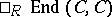# Almost-split sequence

Auslander–Reiten sequence

Roughly speaking, almost-split sequences are minimal non-split short exact sequences. They were introduced by M. Auslander and I. Reiten in 1974–1975 and have become a central tool in the theory of representations of finite-dimensional algebras (cf. also Representation of an associative algebra).

Let $R$ be an Artin algebra, i.e. $R$ is an associative ring with unity that is finitely generated as a module over its centre $Z ( R )$, which is a commutative Artinian ring.

Let $C$ be an indecomposable non-projective finitely-generated left $R$-module. Then there exists a short exact sequence

\begin{equation} \tag{a1} 0 \rightarrow A \stackrel { f } { \rightarrow } B \stackrel { g } { \rightarrow } C \rightarrow 0 \end{equation}

in $\square _ { R } \ \operatorname{Mod}$, the category of finitely-generated left $R$-modules, with the following properties:

i) $A$ and $C$ are indecomposable;

ii) the sequence does not split, i.e. there is no section $s : C \rightarrow B$ of $g$ (a homomorphism such that $g s = \operatorname{id}$), or, equivalently, there is no retraction of $f$ (a homomorphism $r : B \rightarrow A$ such that $r f = \operatorname{id}$);

iii) given any $h : Z \rightarrow C$ with $Z$ indecomposable and $h$ not an isomorphism, there is a lift of $h$ to $B$ (i.e. a homomorphism $\tilde { h } : Z \rightarrow B$ in $\square _ { R } \ \operatorname{Mod}$ such that $g \tilde { h } = h$);

iv) given any $j : A \rightarrow X$ with $X$ indecomposable and $j$ not an isomorphism, there is a homomorphism $\tilde { j } : B \rightarrow X$ such that $\tilde { j } f = j$.

Note that if iii) (or, equivalently, iv)) were to hold for all $h$, not just those $h$ that are not isomorphisms, the sequence (a1) would be split, whence "almost split" . Moreover, a sequence (a1) with these properties is uniquely determined (up to isomorphism) by $C$, and also by $A$. This is the basic Auslander–Reiten theorem on almost-split sequences, [a1], [a8], [a9], [a10], [a11].

For convenience (things also work more generally), let now $R$ be a finite-dimensional algebra over an algebraically closed field $k$. The category $\square _ { R } \ \operatorname{Mod}$ is a Krull–Schmidt category (Krull–Remak–Schmidt category), i.e. a $C \in \square _ { R } \operatorname{Mod}$ is indecomposable if and only if, the endomorphism ring of $C$, is a local ring and (hence) the decomposition of a module in $\square _ { R } \ \operatorname{Mod}$ into indecomposables is unique up to isomorphism.

Let $C$ be an indecomposable and consider the contravariant functor $X \mapsto \square _ { R } \operatorname { Mod } ( X , C )$. The morphisms $g : X \rightarrow C$ that do not admit a section (i.e. an $s : C \rightarrow X$ such that $g s = \operatorname{id}$) form a vector subspace $E _ { C } ( X ) \subset \square _ { R } \operatorname { Mod } ( X , C )$. Let $S _ { C }$ be the quotient functor $S _ { C } = \operatorname { Mod } ( ? , C ) / E _ { C }$. Then, for an indecomposable $D$, $S _ { C } ( D ) = k$ if $D$ is isomorphic to $C$ and zero otherwise. So $S _ { C }$ is a simple functor. (All functors $\Box_R \text { Mod } ( ? , C )$, $E _ { C }$, $S _ { C }$ are viewed as $k$-functors, i.e. functors that take their values in the category of vector $k$-spaces.) If $C$ is indecomposable, then (the Auslander–Reiten theorem, [a4], p.4) the simple functor $S _ { C }$ admits a minimal projective resolution of the form

\begin{equation*} 0 \rightarrow \square _ { R } \operatorname { Mod } ( ? , A ) \rightarrow \square _ { R } \operatorname { Mod } ( ? , B ) \rightarrow \end{equation*}

\begin{equation*} \rightarrow \square _ { R } \text { Mod } ( ? , C ) \rightarrow S _ { C } \rightarrow 0. \end{equation*}

If $C$ is projective, $A$ is zero, otherwise $A$ is indecomposable.

If $C$ is not projective, the sequence $0 \rightarrow A \rightarrow B \rightarrow C \rightarrow 0$ is exact and is the almost-split sequence determined by $C$.

This functorial definition is used in [a5] in the somewhat more general setting of exact categories.

For a good introduction to the use of almost-split sequences, see [a6]; see also [a3], [a5] for comprehensive treatments. See also Riedtmann classification for the use of almost-split sequences and the Auslander–Reiten quiver in the classification of self-injective algebras.

The Bautista–Brunner theorem says that if $R$ is of finite representation type and $0 \rightarrow A \rightarrow B \rightarrow C \rightarrow 0$ is an almost-split sequence, then $B$ has at most $4$ terms in its decomposition into indecomposables; also, if there are indeed $4$, then one of these is projective-injective. This can be generalized, [a7].

How to Cite This Entry:
Almost-split sequence. Encyclopedia of Mathematics. URL: http://encyclopediaofmath.org/index.php?title=Almost-split_sequence&oldid=50571
This article was adapted from an original article by M. Hazewinkel (originator), which appeared in Encyclopedia of Mathematics - ISBN 1402006098. See original article